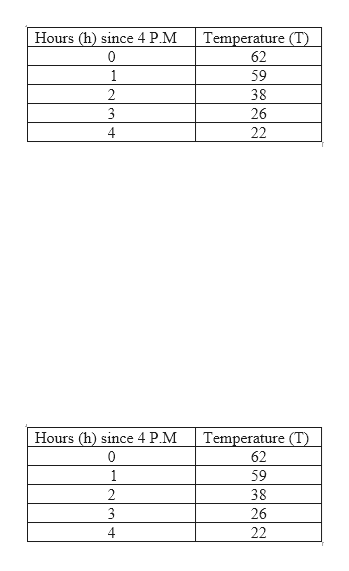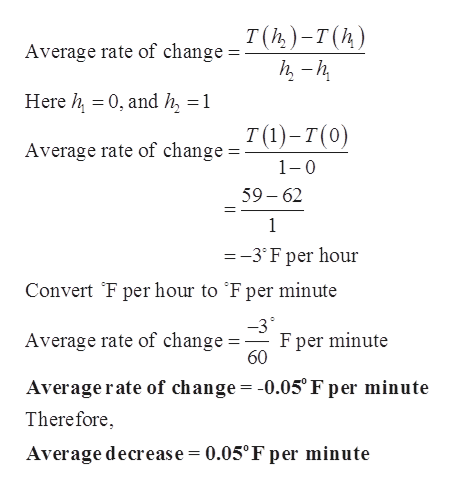# At 4 p.m. on a winter day, an arctic air mass moved from Kansas into Oklahoma, causing temperatures to plummet. Thetemperature T = T(h) in degrees Fahrenheit h hours after 4 p.m. in Stillwater, Oklahoma, on that day is recorded in the followingtable.HoursT Temperaturesince 4 P.M.620591382263224WarmCold(a) Use functional notation to express the temperature in Stillwater at 5:30 p.mXEstimate the value of the above term. (Round your answer to one decimal place.)OX(b) What was the average rate of change per minute in temperature between 4 p.m. and 5 p.m.? (Round your answer totwo decimal places.)XWhat was the average decrease per minute over that time interval? (Round your answer to two decimal places.)X /min(c) Estimate the temperature at 4:15 p.m. (Round your answer to one decimal place.)X(d) At about what time did the temperature reach the freezing point?630p.mExplain your reasoning.

Question
79 views

What are the blanks?

check_circle

Step 1

The temperature T in Fahrenheit after the time 4 P.M. in hours is shown in the below table.help_outlineImage TranscriptioncloseTemperature (T) Hours (h) since 4 P.M 0 62 1 59 2 38 26 4 22 Temperature (T) Hours (h) since 4 P.M 0 62 1 59 2 38 3 26 22 fullscreen
Step 2

The temperature in the still water is given by T(1.5) and the estimate value of it is obtained by taking average as shown.

Step 3

The average rate of change between two ...help_outlineImage TranscriptioncloseT(h)-т(п) Average rate of change h-h Here h 0, and h 1 T()-т(0) Average rate of change = 1 0 59-62 1 =-3°Fper hour Convert F per hour to 'F per minute F per minute 60 Average rate of change = Averagerate of change = -0.05° F per minute Therefore Average decrease = 0.05°F per minute fullscreen

### Want to see the full answer?

See Solution

#### Want to see this answer and more?

Solutions are written by subject experts who are available 24/7. Questions are typically answered within 1 hour.*

See Solution
*Response times may vary by subject and question.
Tagged in

### Other